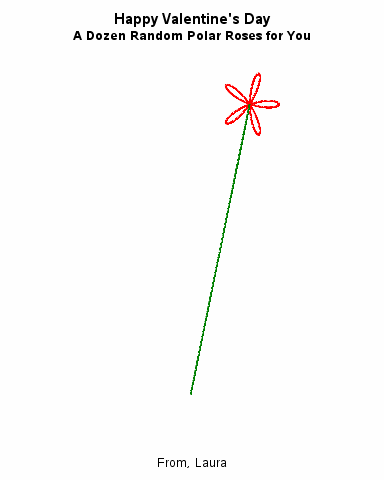## Happy Valentines Day: A Dozen Polar Roses

I was inspired by the blog post, http://blogs.sas.com/content/iml/2015/12/16/polar-rose.html  to make a beautiful Valentine with SAS.  I was thinking as well you could also make a data set with people's names and use by group processing to make personalized Valentine's but for now you can see below the basic idea.  I had fun make these.  The roses are random, so each time you run the macro you get slight differences in the selection of roses.``````/* rose picture*/
ods graphics/reset=all;
ods graphics/ imagefmt=png;
options device=png;

options nodate nonumber;

*ods graphics/ height=6.5in noborder;
ods pdf gtitle gfootnote
file="n:\rose_card.pdf"
startpage=no ;
title;
ODS USEGOPT ;
%macro makecard(for=Todd,from=Mom);

data Roses;
/* the array below has a list of multipliers that make
pretty roses, it is temporary, so will not be saved
with the data*/
array klist{11} _temporary_ (4, 5, 6, 1.5, 2.5, 1.333333, 2.33333, .75, 1.25, 1.2, .8333333);

do flower=1 to 12;
n = ceil( 11*rand("Uniform") );

/*pick a random number
between 1 and 11*/
k=klist{n}; /*assign k to be the nth multiplier*/

/* draw the rose r=cos(k * theta) */
do theta = 0 to 12*constant("pi") by 0.1;
r = cos(k * theta);      /* generalized rose */
x = r*cos(theta);        /* convert to Euclidean coords */
y = r*sin(theta);

/*move the rose to the right spot*/
if flower <= 5 then
do;
cx=2*flower+1;
cy=9;
end;
else if 6<= flower<=9 then
do;
cx=(2*flower-8);
cy=10.5;
end;
else if 10<= flower<=12 then
do;
cx=(2*flower-15);
cy=12;
end;

x=x+cx;
y=y+cy;
group=flower;
output;

/*make the stem*/
group=-flower;
x=cx;
y=cy;
output;
x=7;
y=3;
output;
end;
end;

/*bow*/
do theta = constant("pi")*7.5/12 to constant("pi")*28.5/12 by 0.01;
r = cos(2 * (theta));       /* rose */
x = r*cos(theta);          /* convert to Euclidean coords */
y = r*sin(theta);
group=100;

if y<abs(x) then
do;
x=x+7;
y=y+3;
output;
end;
end;
run;

proc sort data=roses;
by group;
title1 "Happy Valentines Day &for" ;
title2 "A Dozen Random Polar Roses for You" ;

proc sgplot data=Roses aspect=1 noautolegend
noborder nowall;
styleattrs datacontrastcolors=
(
green green green green
green green green green
green green green green
red  bippk red purple bippk
blue purple  bippk red purple  blue red
crimson
) datalinepatterns=(1);
series x=x y=y /group=group;
xaxis min=0 max=15 display=none;
yaxis min=0 max=15 display=none;
footnote "Lots of Love, &from";
footnote2 " ";
footnote3 " ";
footnote4 " ";
footnote5 " Polar Roses: r = cos(k*theta)";
footnote6 " Generated with the SAS System 9.4";
footnote7 "initial idea: http://blogs.sas.com/content/iml/2015/12/16/polar-rose.html";
run;
title;
footnote;

%mend;

%makecard(for=Todd ,from=Laura);

ods pdf close;``````

3 REPLIES 3

## Re: Happy Valentines Day: A Dozen Polar Roses

This post *totally* makes my day, @LauraRK! Love what @Rick_SAS did for Valentine's Day 2015: https://communities.sas.com/t5/SAS-Analytics-U/Give-A-Geeky-Valentine/m-p/164135/highlight/true#M806

Check out the SAS Explore forum. You'll find posts summarizing more than 100 presentations.

## Re: Happy Valentines Day: A Dozen Polar Roses

Happy Valentines Day, just bumping this up, as it is that time of year again.

## Re: Happy Valentines Day: A Dozen Polar Roses``````/* rose picture with animation*/
%let for=You;
%let from=Laura;
title;
footnote;
%let outpath=W:\valentines;

ods graphics / imagefmt=GIF width=4in height=5in;     /* each image is 4in x 5in GIF */
options papersize=('4 in', '5 in')                    /* set size for images */
nodate nonumber                               /* do not show date, time, or frame number */
animduration=0.5 animloop=yes noanimoverlay   /* animation details */
printerpath=gif animation=start;              /* start recording images to GIF */
ods printer file="&outpath\Anim.gif";  /* images saved into animated GIF */

%macro makedata;
data Roses;
/* the array below has a list of multipliers that make
pretty roses, it is temporary, so will not be saved
with the data*/
array klist{11} _temporary_ (4, 5, 6, 1.5, 2.5, 1.333333, 2.33333, .75, 1.25, 1.2, .8333333);

do flower=1 to 12;
n = ceil( 11*rand("Uniform") );
/*pick a random number
between 1 and 11*/
k=klist{n}; /*assign k to be the nth multiplier*/

/* draw the rose r=cos(k * theta) */
do theta = 0 to 12*constant("pi") by 0.1;
r = cos(k * theta);      /* generalized rose */
x = r*cos(theta);        /* convert to Euclidean coords */
y = r*sin(theta);

/*move the rose to the right spot*/
if flower <= 5 then
do;
cx=2*flower+1;
cy=9;
end;
else if 6<= flower<=9 then
do;
cx=(2*flower-8);
cy=10.5;
end;
else if 10<= flower<=12 then
do;
cx=(2*flower-15);
cy=12;
end;

x=x+cx;
y=y+cy;
group=flower;
do i=0 to flower; output; end;

/*make the stem*/
group=flower+.5;
x=cx;
y=cy;
do i=0 to flower; output; end;
x=7;
y=3;
do i= 0 to flower; output; end;
end;
end;

/*bow*/
do theta = constant("pi")*7.5/12 to constant("pi")*28.5/12 by 0.01;
r = cos(2 *(theta));       /* rose */
x = r*cos(theta);          /* convert to Euclidean coords */
y = r*sin(theta);
group=100;
i=0;
if y<abs(x) then
do;
x=x+7;
y=y+3;
output;
end;
end;
run;

%mend;

%makedata;
data roses2 ;
set roses;
i=13-i;
run;

proc sort data=roses2;
by i group;
run;

options nobyline;
proc sgplot data=Roses2  aspect=1 noautolegend
noborder nowall noopaque;;
by i;
styleattrs datacontrastcolors=
(
red green
bippk green red green purple green bippk green
blue green purple green  bippk green red green purple green
blue green red green
crimson
) datalinepatterns=(1);
series x=x y=y /group=group;
xaxis min=1 max=13 display=none;
yaxis min=2 max=13 display=none;
title1 "Happy Valentine's Day";
title2 "A Dozen Random Polar Roses for &for";
footnote1 "From, &from";
/*	footnote2 " Polar Roses: r = cos(k*theta)";
footnote3 " Generated with the SAS System 9.4";
footnote4 "see: http://blogs.sas.com/content/iml/2015/12/16/polar-rose.html";
*/
run;
/* restore screen output */

options printerpath=gif animation=stop;               /* stop recording images */
ods printer close;

title;
footnote;``````
Discussion stats
• 3 replies
• 2601 views
• 13 likes
• 2 in conversation